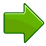# Balance when Adding and Subtracting

Algebra is great fun - you get to solve puzzles!

When we add or subtract the same thing from both sides the equation stays in balance.

Watch the equation below and try to get "x = (something)" using the +x, −x, +1, −1 buttons.

images/balance.js

## Things to Try

Each "equation" will have a solution like "x=2" (or maybe "x=3", etc). Try to see all the different ways this answer can be hidden.First, try to solve the equation by adding and subtracting until you get an answer like x = (value)Then add and subtract some more until you get x - (value) = 0Then try to get a solution like - (value) = -xThen just add and subtract different amounts to see all the possibilities. Now press "New Equation" and try out some more ... !